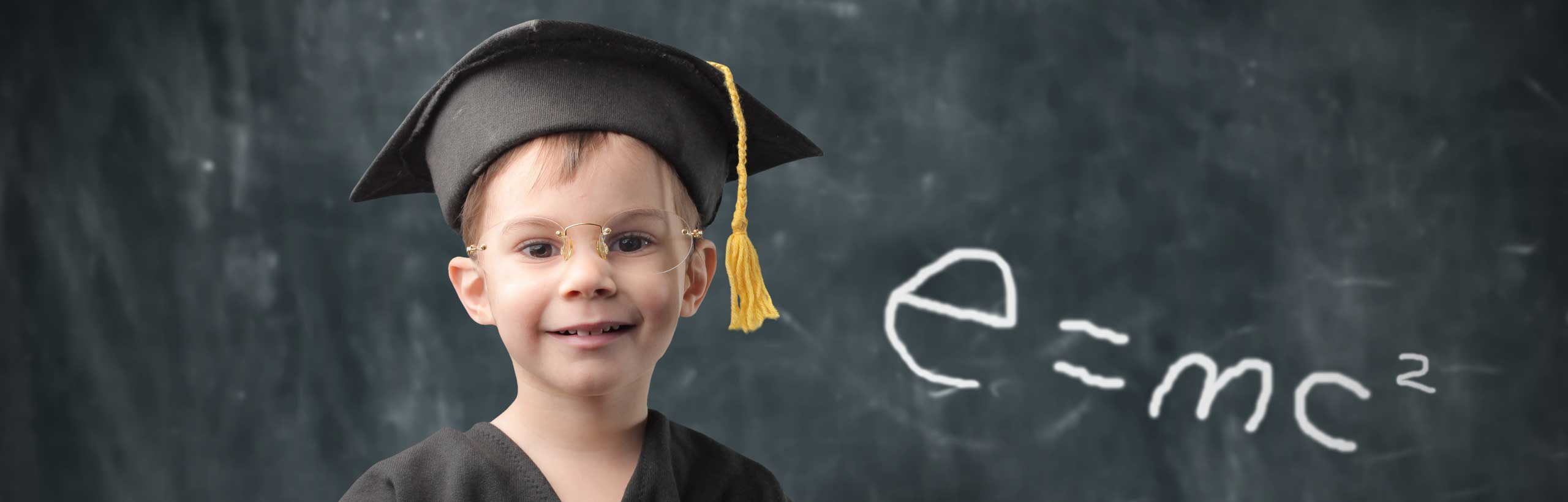# Intermediate Algebra Problems With Answers - Sample 2

A set of intermediate algebra problems on lines, slope, functions, domain, range , x and y intercepts ... , with answers, are presented. The solutions are at the bottom of the page.

 Line L passes through the points (1,3) and (-3,4). a) Fine the slope of line L. b) Find the y-intercept of L. c) Find the x-intercept of L. d) Find b so that line L passes through the point (-4,b). The graph of function f is shown below. a) Find the domain of f. b) Find the range of f. c) Find f(0), f(1) and f(2). d) Find all values of x for which f(x) = 5.. The graph of line segment AB is shown below. a) Use the graph to find the coordinates of points A and B. b) Find the length of the line segment AB. c) Find the midpoint of the line segment AB.. Line L1 passes through the point (1 , b) as shown below. Find the equation of line L2 that passes through point A and is perpendicular to line L1.. Are the two lines L1 through the points (-1,4) and (0,4) and L2 through the points (5,6) and (5,2) parallel, perpendicular or neither. Explain your answer. Find all values of the constant K so that the inequality 5(x - K + 2) ≥ 2(x + 4) - 7 has a solution set given by the interval [10 , + infinity). Linda walked for 2 hours then ran for 1 hour. If she runs three times as fast as she walks and the total trip was 20 kilometers, then how fast does she run? Each pair of the three lines defined by their equations y = (1/2) x - 1, y = 2x + 2 and y = -x + 2 has a point of intersection so that when put together there are three points of intersection making a triangle ABC. Show that triangle ABC is isosceles. Answers to the Above Questions a) slope = (4 - 3) / (-3 - 1) = -1/4 b) Equation of line L: y - 3 = (-1/4)(x - 1) To find the y-intercept, put x = 0 in the equation: y - 3 = 1/4 , y = 13/4 c) To find the x-intercept, put y = 0 in the equation: 0 - 3 = (-1/4)(x - 1) , x = 13 d) Substitute x and y by -4 and b respectively in the equation of line L and solve for b. b - 3 = (-1/4)(-4 - 1) , b = 17/4 a) Domain: (-4 , 4] b) Range: [-4 , 12] c) f(0) = -4, f(1) = -3, f(2) = 0 d) f(x) = 5 for x = -3 or x = 3 a) A(-2 , 4) , B(4 , 2) b) length of AB = √[ (2 - 4) 2 + (2 - 4) 2 ] = 2√10 c) Midpoint coordinates: x = (-2+4)/2 = 1 , y = (4+2)/2 = 3 From graph, x-intercept (-3 , 0), y-intercept (0 , 2) Slope of L1 = (2 - 0) / (0 - (-3)) = 2/3 Find b: slope using the y-intercept and point (1 , b) (b - 2)/(1 - 0) = 2/3 solve to find b = 8/3 Slope of L2 = - 1/slope of L1 = -3/2 (relationship between slopes of perpendicular lines) equation of L2: y - 8/3 = -3/2 (x - 1) or y = (-3/2) x + 25/6 Slope of L1 = (4 - 4) / (0 + 1) = 0 , L1 is horizontal Slope of L2 = (6 - 2) / (5 - 5) = undefined , L2 is vertical The two lines are perpendicular. Solve the given inequality 5(x - K + 2) ≥ 2(x + 4) - 7 to obtain the solution set x ≥ -3 + 5K/3 For the above set to be equal to the set [10 , +infinity) we need to have -3 + 5K/3 = 10 Solve the above for K to obtain: K = 39/5 Let x be the walking speed. 3x will be the running speed. 2*(x) will be the distance walked and 1*(3x) will be the distance run. Total distance is 20 km. Hence 2x + 3x = 20 x = 4 km/hr , 3x = 12 km/hr and it is the speed of Linda when running. let A be the point of intersection of the lines y = (1/2) x - 1 and y = 2x + 2. Solve the system of equations made by the equations of the two lines to find the point. (1/2) x - 1 = 2x + 2 , solve for x to find x = -2 and substitute to find y = -2. Hence point A has coordinates (-2 , -2). Let point B be the point of intersection of the lines y = (1/2) x - 1 and y = -x + 2. using the same ideas as above to find the coordinates of B as (2 , 0). Let point C be the point of intersection of the lines y = -x + 2 and y = 2x + 2. And similar ideas can be used to find the coordinates of C as (0 , 2). length of line segment AB = √(2 2 + 4 2) = 2√5 length of line segment AC = √(4 2 + 2 2) = 2√5 length of line segment BC = √(2 2 + 2 2) = 2√2 length of AB = length of AC and therefore the triangle is isosceles. Algebra Questions and problems More ACT, SAT and Compass practice